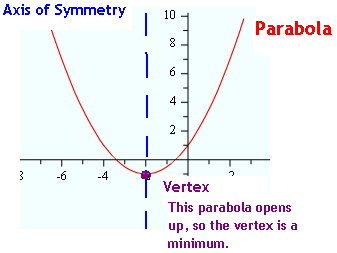Introduction

In general the graph of a quadratic equation, y = ax2 + bx + c, is called a parabola which is a U-shaped graph. The axis of symmetry is a vertical line through the vertex of the parabola. All parabolas are symmetric with respect to their axis of symmetry. The vertex of a parabola will be either the minimum or maximum point of the parabola, depending on whether the graph opens upward or downward.